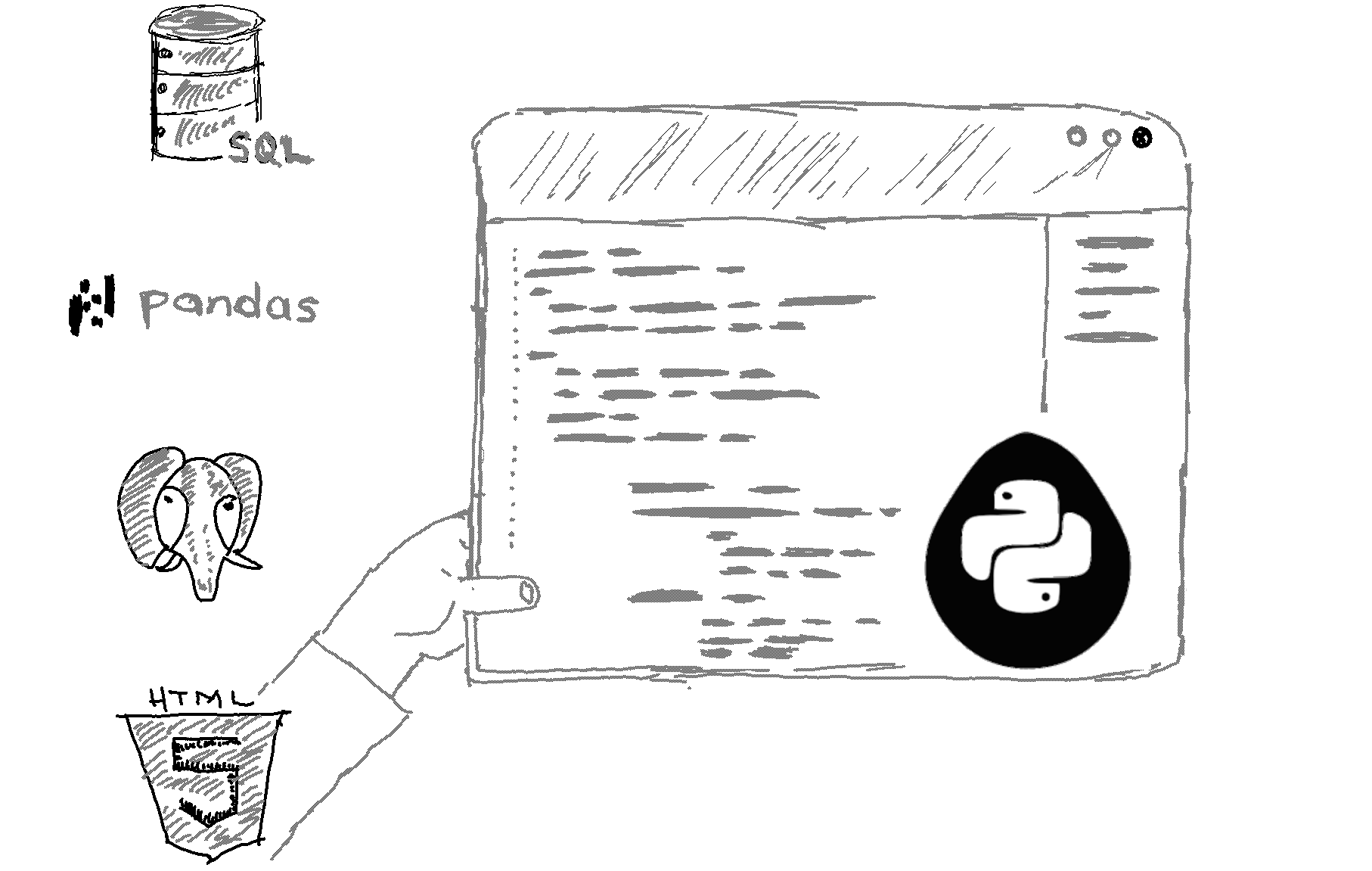# Here is how to check if a list is empty in Python.

```# Define a list
my_list = []

# Check if the list is empty
if len(my_list) == 0:
print("The list is empty.")
else:
print("The list is not empty.") ```

Output
`The list is empty.`

Explanation

To check if a list is empty in Python, you can use the len() function to check the length of the list. If the length is 0, it means the list is empty.

Alternatively, you can use the bool() function to check if a list is empty. This function returns False if the list is empty, and True otherwise. Here's an example:

```# Define a list
my_list = []

# Check if the list is empty
if not bool(my_list):
print("The list is empty.")
else:
print("The list is not empty.")```
This will also print 'The list is empty'.

*Note that both of these methods will work for any iterable object, not just lists. So you can use them to check if any iterable object, such as a tuple or a set, is empty.

Related HowTos
##### Recommended Course

Learn Flask development and learn to build cool apps with our premium Python course on Udemy.